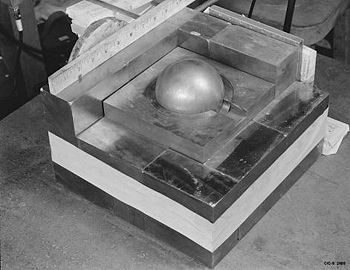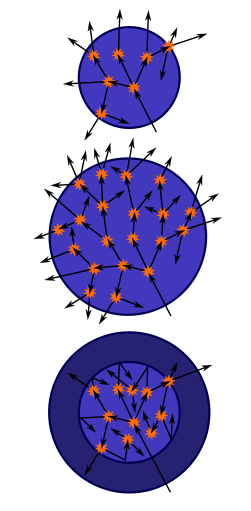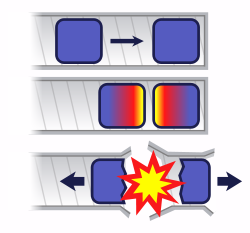# Critical mass

﻿
Critical massAs part of a re-creation of a 1945 criticality accident, a plutonium pit is surrounded by blocks of neutron-reflective tungsten carbide. The original experiment was designed to measure the radiation produced when an extra block was added. Instead, the mass went supercritical.

A critical mass is the smallest amount of fissile material needed for a sustained nuclear chain reaction. The critical mass of a fissionable material depends upon its nuclear properties (e.g. the nuclear fission cross-section), its density, its shape, its enrichment, its purity, its temperature and its surroundings.

## Explanation of criticality

When a nuclear chain reaction in a mass of fissile material is self-sustaining, the mass is said to be in a critical state in which there is no increase or decrease in power, temperature or neutron population.

A numerical measure of a critical mass is dependent on the effective neutron multiplication factor k, the average number of neutrons released per fission event that go on to cause another fission event rather than being absorbed or leaving the material. When k = 1, the mass is critical, and the chain reaction is barely self-sustaining.

A subcritical mass is a mass of fissile material that does not have the ability to sustain a fission chain reaction. A population of neutrons introduced to a subcritical assembly will exponentially decrease. In this case, k < 1. A steady rate of spontaneous fissions causes a proportionally steady level of neutron activity. The constant of proportionality increases as k increases.

A supercritical mass is one where there is an increasing rate of fission. The material may settle into equilibrium (i.e. become critical again) at an elevated temperature/power level or destroy itself, by which equilibrium is reached. In the case of supercriticality, k > 1.

## Changing the point of criticality

The point and therefore the mass where criticality occurs may be changed by modifying certain attributes such as fuel, shape, temperature, density and the installation of a neutron-reflective substance. These attributes have complex interactions and interdependencies. This section explains only the simplest ideal cases.

• Varying the amount of fuel

It is possible for a fuel assembly to be critical at near zero power. If the perfect quantity of fuel were added to a slightly subcritical mass to create an "exactly critical mass", fission would be self-sustaining for one neutron generation (fuel consumption makes the assembly subcritical).

If the perfect quantity of fuel were added to a slightly subcritical mass, to create a barely supercritical mass, the temperature of the assembly would increase to an initial maximum (for example: 1 K above the ambient temperature) and then decrease back to room temperature after a period of time, because fuel consumed during fission brings the assembly back to subcriticality once again.

• Changing the shape

A mass may be exactly critical without being a perfect homogeneous sphere. More closely refining the shape toward a perfect sphere will make the mass supercritical. Conversely changing the shape to a less perfect sphere will decrease its reactivity and make it subcritical.

• Changing the temperature

A mass may be exactly critical at a particular temperature. Fission and absorption cross-sections increase as the relative neutron velocity decreases. As fuel temperature increases, neutrons of a given energy appear faster and thus fission/absorption is less likely. This is not unrelated to doppler broadening of the U238 resonances but is common to all fuels/absorbers/configurations. Neglecting the very important resonances, the total neutron cross section of every material exhibits an inverse relationship with relative neutron velocity. Hot fuel is always less reactive than cold fuel (over/under moderation in LWR is a different topic). Thermal expansion associated with temperature increase also contributes a negative coefficient of reactivity since fuel atoms are moving farther apart. A mass that is exactly critical at room temperature would be sub-critical in an environment anywhere above room temperature due to thermal expansion alone.

• Varying the density of the mass

The higher the density, the lower the critical mass. The density of a material at a constant temperature can be changed by varying the pressure or tension or by changing crystal structure (see Allotropes of plutonium). An ideal mass will become subcritical if allowed to expand or conversely the same mass will become supercritical if compressed. Changing the temperature may also change the density; however, the effect on critical mass is then complicated by temperature effects (See Changing the temperature) and by whether the material expands or contracts with increased temperature. Assuming the material expands with temperature (enriched Uranium 235 at room temperature for example), at an exactly critical state, it will become subcritical if warmed to lower density or become supercritical if cooled to higher density. Such a material is said to have a negative temperature coefficient of reactivity to indicate that its reactivity decreases when its temperature increases. Using such a material as fuel means fission decreases as the fuel temperature increases.

• Use of a neutron reflector

Surrounding a spherical critical mass with a neutron reflector further reduces the mass needed for criticality. A common material for a neutron reflector is beryllium metal. This reduces the number of neutrons which escape the fissile material, resulting in increased reactivity.

• Use of a tamper

In a bomb, a dense shell of material surrounding the fissile core will contain, via inertia, the expanding fissioning material. This increases the efficiency. Because a bomb relies on fast neutrons (not ones moderated by reflection with light elements, as in a reactor) the tamper in a bomb is not functioning as a neutron reflector. Also, if the tamper is (e.g. depleted) uranium, it can fission due to the high energy neutrons generated by the primary explosion. This can greatly increase yield, especially if even more neutrons are generated by fusing hydrogen isotopes, in a so-called boosted configuration.

## Critical mass of a bare sphereTop: A sphere of fissile material is too small to allow the chain reaction to become self-sustaining as neutrons generated by fissions can too easily escape.

Middle: By increasing the mass of the sphere to a critical mass, the reaction can become self-sustaining.

Bottom: Surrounding the original sphere with a neutron reflector increases the efficiency of the reactions and also allows the reaction to become self-sustaining.

The shape with minimal critical mass and the smallest physical dimensions is a sphere. Bare-sphere critical masses at normal density of some actinides are listed in the following table.

Nuclide Critical Mass
(kg)
Diameter
(cm)
Ref
uranium-233 15 11 
uranium-235 52 17 
neptunium-236 7 8.7 
neptunium-237 60 18 
plutonium-238 9.04–10.07 9.5-9.9 
plutonium-239 10 9.9 
plutonium-240 40 15 
plutonium-241 12 10.5 
plutonium-242 75–100 19-21 
americium-241 55–77 20-23 
americium-242 9–14 11-13 
americium-243 180–280 30-35 
curium-243 7.34–10 10-11 
curium-244 (13.5)–30 (12.4)–16 
curium-245 9.41–12.3 11-12 
curium-246 39–70.1 18-21 
curium-247 6.94–7.06 9.9 
californium-249 6 9 
californium-251 5 8.5 
californium-252 2.73 6.9 

The critical mass for lower-grade uranium depends strongly on the grade: with 20% U-235 it is over 400 kg; with 15% U-235, it is well over 600 kg.

The critical mass is inversely proportional to the square of the density. If the density is 1% more and the mass 2% less, then the volume is 3% less and the diameter 1% less. The probability for a neutron per cm travelled to hit a nucleus is proportional to the density. It follows that 1% greater density means that the distance travelled before leaving the system is 1% less. This is something that must be taken into consideration when attempting more precise estimates of critical masses of plutonium isotopes than the approximate values given above, because plutonium metal has a large number of different crystal phases which can have widely varying densities.

Note that not all neutrons contribute to the chain reaction. Some escape and others undergo radiative capture.

Let q denote the probability that a given neutron induces fission in a nucleus. Let us consider only prompt neutrons, and let ν denote the number of prompt neutrons generated in a nuclear fission. For example, ν ≈ 2.5 for uranium-235. Then, criticality occurs when ν·q = 1. The dependence of this upon geometry, mass, and density appears through the factor q.

Given a total interaction cross section σ (typically measured in barns), the mean free path of a prompt neutron is$\ell^{-1} = n \sigma$ where n is the nuclear number density. Most interactions are scattering events, so that a given neutron obeys a random walk until it either escapes from the medium or causes a fission reaction. So long as other loss mechanisms are not significant, then, the radius of a spherical critical mass is rather roughly given by the product of the mean free path$\ell$ and the square root of one plus the number of scattering events per fission event (call this s), since the net distance travelled in a random walk is proportional to the square root of the number of steps:$R_c \simeq \ell \sqrt{s} \simeq \frac{\sqrt{s}}{n \sigma}$

Note again, however, that this is only a rough estimate.

In terms of the total mass M, the nuclear mass m, the density ρ, and a fudge factor f which takes into account geometrical and other effects, criticality corresponds to$1 = \frac{f \sigma}{m \sqrt{s}} \rho^{2/3} M^{1/3}$

which clearly recovers the aforementioned result that critical mass depends inversely on the square of the density.

Alternatively, one may restate this more succinctly in terms of the areal density of mass, Σ:$1 = \frac{f' \sigma}{m \sqrt{s}} \Sigma$

where the factor f has been rewritten as f' to account for the fact that the two values may differ depending upon geometrical effects and how one defines Σ. For example, for a bare solid sphere of Pu-239 criticality is at 320 kg/m2, regardless of density, and for U-235 at 550 kg/m2. In any case, criticality then depends upon a typical neutron "seeing" an amount of nuclei around it such that the areal density of nuclei exceeds a certain threshold.

This is applied in implosion-type nuclear weapons where a spherical mass of fissile material that is substantially less than a critical mass is made supercritical by very rapidly increasing ρ (and thus Σ as well) (see below). Indeed, sophisticated nuclear weapons programs can make a functional device from less material than more primitive weapons programs require.

Aside from the math, there is a simple physical analog that helps explain this result. Consider diesel fumes belched from an exhaust pipe. Initially the fumes appear black, then gradually you are able to see through them without any trouble. This is not because the total scattering cross section of all the soot particles has changed, but because the soot has dispersed. If we consider a transparent cube of length L on a side, filled with soot, then the optical depth of this medium is inversely proportional to the square of L, and therefore proportional to the areal density of soot particles: we can make it easier to see through the imaginary cube just by making the cube larger.

Several uncertainties contribute to the determination of a precise value for critical masses, including (1) detailed knowledge of cross sections, (2) calculation of geometric effects. This latter problem provided significant motivation for the development of the Monte Carlo method in computational physics by Nicholas Metropolis and Stanislaw Ulam. In fact, even for a homogeneous solid sphere, the exact calculation is by no means trivial. Finally note that the calculation can also be performed by assuming a continuum approximation for the neutron transport. This reduces it to a diffusion problem. However, as the typical linear dimensions are not significantly larger than the mean free path, such an approximation is only marginally applicable.

Finally, note that for some idealized geometries, the critical mass might formally be infinite, and other parameters are used to describe criticality. For example, consider an infinite sheet of fissionable material. For any finite thickness, this corresponds to an infinite mass. However, criticality is only achieved once the thickness of this slab exceeds a critical value.

## Criticality in nuclear weapon designIf two pieces of subcritical material are not brought together fast enough, nuclear predetonation (fizzle) can occur, whereby a very small explosion will blow the bulk of the material apart.

Until detonation is desired, a nuclear weapon must be kept subcritical. In the case of a uranium bomb, this can be achieved by keeping the fuel in a number of separate pieces, each below the critical size either because they are too small or unfavorably shaped. To produce detonation, the uranium is brought together rapidly. In Little Boy, this was achieved by firing a piece of uranium (a 'doughnut'), down a gun barrel onto another piece, (a 'spike'), a design referred to as a gun-type fission weapon.

A theoretical 100% pure Pu-239 weapon could also be constructed as a gun-type weapon, like the Manhattan Project's proposed Thin Man design. In reality, this is impractical because even "weapons grade" Pu-239 is contaminated with a small amount of Pu-240, which has a strong propensity toward spontaneous fission. Because of this, a reasonably-sized gun-type weapon would suffer nuclear reaction before the masses of plutonium would be in a position for a full-fledged explosion to occur.

Instead, the plutonium is present as a subcritical sphere (or other shape), which may or may not be hollow. Detonation is produced by exploding a shaped charge surrounding the sphere, increasing the density (and collapsing the cavity, if present) to produce a prompt critical configuration. This is known as an implosion type weapon.

Wikimedia Foundation. 2010.

### Look at other dictionaries:

• Critical Mass — Critical Mass …   Википедия

• critical mass — ˌcritical ˈmass noun [singular] COMMERCE MARKETING the minimum number of users, buyers etc that is necessary for a product to succeed or for something to happen: critical mass of • A communications system is of no value to the users unless there… …   Financial and business terms

• Critical Mass — (englische für kritische Masse) bezeichnet: Kritische Masse in der Kernphysik Critical Mass (Protestform), monatliches Radfahrereignis Critical Mass (Gruppe), niederländische Happy Hardcore Gruppe Critical Mass – Wettlauf mit der Zeit, US… …   Deutsch Wikipedia

• critical mass — n [U and C] 1.) the amount of a substance that is necessary for a ↑nuclear ↑chain reaction to start 2.) the smallest number of people or things that are needed in order for something to happen or be possible ▪ How can we get a critical mass of… …   Dictionary of contemporary English

• critical mass — [n] crisis point critical point, critical stage, crossroads*, crunch time, do or die time*, high noon*, irreversible momentum, moment of truth, point of no return, sink or swim time*, the Rubicon, turning point; concept 674 …   New thesaurus

• Critical mass — См. Критическая масса Термины атомной энергетики. Концерн Росэнергоатом, 2010 …   Термины атомной энергетики

• critical mass — noun 1. ) count or uncount SCIENCE the smallest amount of a substance needed to cause a nuclear reaction 2. ) singular or uncount the smallest number of people or things needed to make something happen …   Usage of the words and phrases in modern English

• critical mass — The minimum amount of resources or number of people needed to start and/or sustain a business, project or event …   The small dictionary of idiomes

• critical mass — n. 1. the minimum amount of fissile material that can sustain a nuclear chain reaction under a given set of conditions 2. the minimum amount or number required for something to happen, begin, etc …   English World dictionary

• Critical Mass — This article is about bicycle events. For the nuclear reaction, see Critical mass. For other uses of the term critical mass , see Critical mass (disambiguation). San Francisco Critical Mass, April 29, 2005. Critical Mass is a cycling event… …   Wikipedia